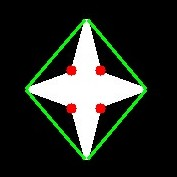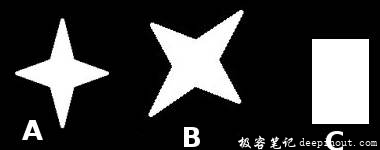# OpenCV-Python更多轮廓功能

• 凸性缺陷以及如何找到它们。
• 查找点到多边形的最短距离
• 匹配不同的形状

## 理论和代码

### 凸包缺陷

OpenCV带有一个现成的函数 cv.convexityDefect() 来查找该函数。基本的函数调用如下所示：

hull = cv.convexHull(cnt,returnPoints = False)
defects = cv.convexityDefects(cnt,hull)


import cv2 as cv
import numpy as np
img_gray = cv.cvtColor(img,cv.COLOR_BGR2GRAY)
ret,thresh = cv.threshold(img_gray, 127, 255,0)
contours,hierarchy = cv.findContours(thresh,2,1)
cnt = contours
hull = cv.convexHull(cnt,returnPoints = False)
defects = cv.convexityDefects(cnt,hull)
for i in range(defects.shape):
s,e,f,d = defects[i,0]
start = tuple(cnt[s])
end = tuple(cnt[e])
far = tuple(cnt[f])
cv.line(img,start,end,[0,255,0],2)
cv.circle(img,far,5,[0,0,255],-1)
cv.imshow('img',img)
cv.waitKey(0)
cv.destroyAllWindows()### 点多边形测试

dist = cv.pointPolygonTest(cnt,(50,50),True)


### 匹配形状

OpenCV带有函数 cv.matchShapes()，使我们能够比较两个形状或两个轮廓，并返回显示相似性的度量。结果越低，匹配越好。它是基于 hu-moment 值计算的。文档中介绍了不同的测量方法。

import cv2 as cv
import numpy as np
ret, thresh = cv.threshold(img1, 127, 255,0)
ret, thresh2 = cv.threshold(img2, 127, 255,0)
contours,hierarchy = cv.findContours(thresh,2,1)
cnt1 = contours
contours,hierarchy = cv.findContours(thresh2,2,1)
cnt2 = contours
ret = cv.matchShapes(cnt1,cnt2,1,0.0)
print( ret )• 匹配图像A本身= 0.0
• 将图像A与图像B匹配= 0.001946
• 将图像A与图像C匹配= 0.326911
看，即使图像旋转也不会对该比较产生太大影响。

Hu-Moments是平移，旋转和缩放不变的七个矩。第七个是偏斜不变的。这些值可以使用 cv.HuMoments() 函数找到。

• 回顶